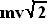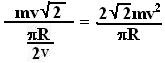## Wednesday, September 5, 2007

### Irodov Problem 1.83a) The average force is change in momentum divided by the total time. Initially the momentum of the particle was mv directed North as shown in th figure. In the end it became mv directed along west. The change in momentum is given by the vector difference between the two vectors and is given by,as shown in the figure. The distance traveled by the particle is one fourth of the circumference and is given by. The time taken to travel this distance is thus,. The force is given by,b)In this case the initial momentum was zero. Thus the change in momentum will be mv. Suppose that it took t units of time to travel the quarter circle, then the average force is mv/t but v/t is the tangential acceleration of the body. Thus, the average force is.